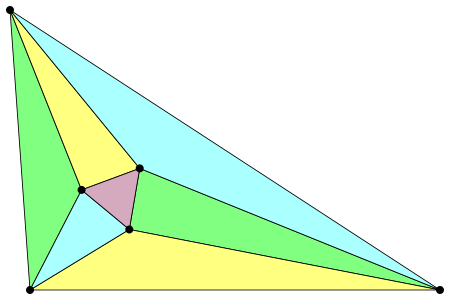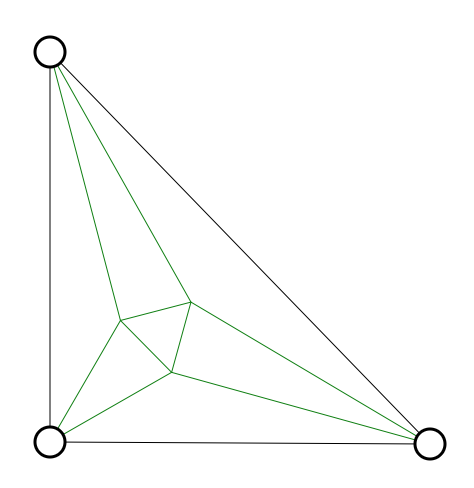# Area of Morley's Triangle

Geometry Level 5The image above is an illustration of what is known as Morley's Theorem. It states that if the angles of any triangle are trisected and the lines extending from these trisections are connected as shown above, then the triangle made by those connections will be equilateral. Such equilateral triangles are known as First Morley Triangles. Observe the following image:The triangle shown above is a 45-45-90 triangle with a hypotenuse of length 4. If the area of the First Morley Triangle depicted in this image can be written as $a\sqrt{b}-c$, where $a$, $b$, and $c$ are positive integers and $b$ is square-free, what is $a+b+c$?

×